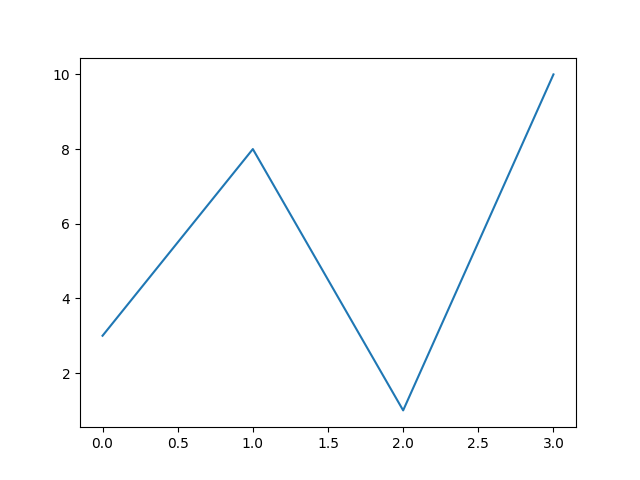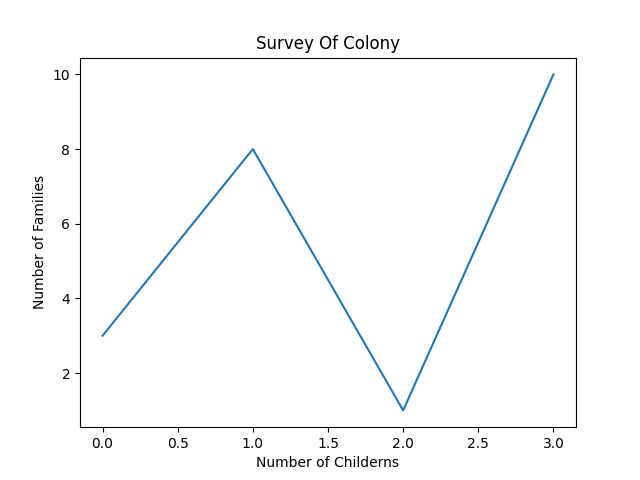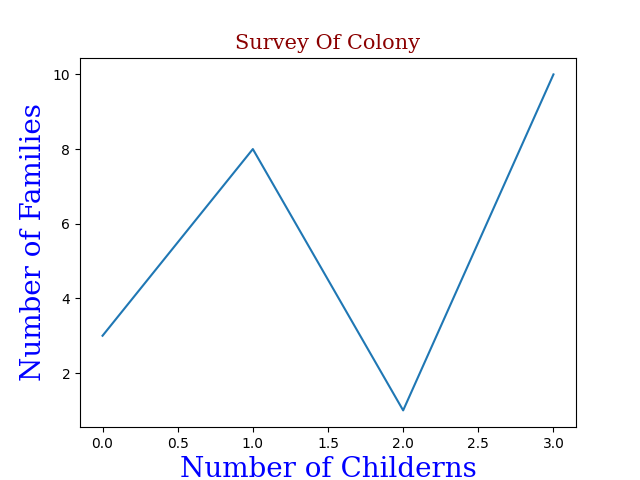Skip to content
Related Articles
How to Add Labels in a Plot using Python?
• Difficulty Level : Medium
• Last Updated : 17 Mar, 2021

Prerequisites: Python Matplotlib

In this article, we will discuss adding labels to the plot using Matplotlib in Python. But first, understand what are labels in a plot. The heading or sub-heading written at the vertical axis (say Y-axis) and the horizontal axis(say X-axis) improves the quality of understanding of plotted stats.

Example: Let’s create a simple plot

## Python

 `# python program to plot graph without labels``import` `matplotlib``import` `matplotlib.pyplot as plt``import` `numpy as np`` ` ` ` `# it will take x coordinates by default ``# starting from 0,1,2,3,4...``y ``=` `np.array([``3``, ``8``, ``1``, ``10``])`` ` `plt.plot(y)``plt.show()`

Output:Plot without Labels or Title

## Creating Labels for a Plot

By using pyplot() function of library we can add xlabel() and ylabel() to set x and y labels.

Example: Let’s add Label in the above Plot

## Python

 `# python program for plots with label``import` `matplotlib``import` `matplotlib.pyplot as plt``import` `numpy as np`` ` ` ` `# Number of chidern it was default in earlier case``x ``=` `np.array([``0``, ``1``, ``2``, ``3``])`` ` `# Number of families``y ``=` `np.array([``3``, ``8``, ``1``, ``10``])`` ` `plt.plot(x, y)`` ` `# Label for x-axis``plt.xlabel(``"Number of Childerns"``)`` ` `# Label for y-axis``plt.ylabel(``"Number of Families"``)`` ` `plt.show()  ``# for display`

Output:Plot with Labels

If you would like to make it more understandable, add a Title to the plot, by just adding a single line of code.

`plt.title("Survey Of Colony")`

Example:

## Python3

 `# python program for plots with label``import` `matplotlib``import` `matplotlib.pyplot as plt``import` `numpy as np`` ` ` ` `# Number of chidern it was default in earlier case``x ``=` `np.array([``0``, ``1``, ``2``, ``3``])`` ` `# Number of families``y ``=` `np.array([``3``, ``8``, ``1``, ``10``])`` ` `plt.plot(x, y)`` ` `# Label for x-axis``plt.xlabel(``"Number of Childerns"``)`` ` `# Label for y-axis``plt.ylabel(``"Number of Families"``)`` ` `# title of the plot``plt.title(``"Survey Of Colony"``)`` ` `plt.show()  ``# for display`

Output:Plot with Title

## Set Font Properties for Titles and Labels

In order to make the Plot more attractive use the fontdict parameter in xlabel(), ylabel(), and title() to apply the font properties.

## Python

 `# Adding font properties to labels and titles``import` `matplotlib``import` `matplotlib.pyplot as plt``import` `numpy as np`` ` ` ` `# Number of Childerns``x ``=` `np.array([``0``, ``1``, ``2``, ``3``])`` ` `# Number of Families``y ``=` `np.array([``3``, ``8``, ``1``, ``10``])`` ` `# label including this form1 will have these properties``form1 ``=` `{``'family'``: ``'serif'``, ``'color'``: ``'blue'``, ``'size'``: ``20``}`` ` `# label including this form2 will have these properties``form2 ``=` `{``'family'``: ``'serif'``, ``'color'``: ``'darkred'``, ``'size'``: ``15``}`` ` `plt.plot(x, y)``plt.xlabel(``"Number of Childerns"``, fontdict``=``form1)``plt.ylabel(``"Number of Families"``, fontdict``=``form1)``plt.title(``"Survey Of Colony"``, fontdict``=``form2)``plt.show()`

Output:Plot with Font Properties

Attention geek! Strengthen your foundations with the Python Programming Foundation Course and learn the basics.

To begin with, your interview preparations Enhance your Data Structures concepts with the Python DS Course. And to begin with your Machine Learning Journey, join the Machine Learning – Basic Level Course

My Personal Notes arrow_drop_up